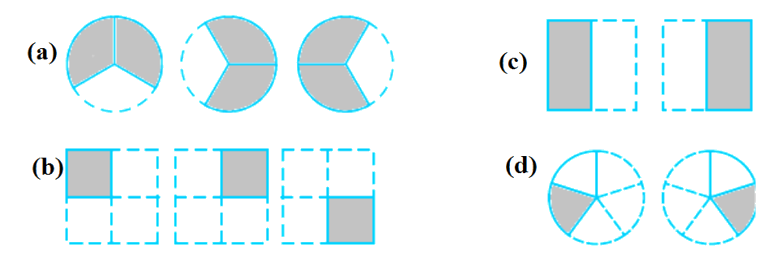# Ex 2.2 Q1 Fractions-and-Decimals-Solutions NCERT Maths Class 7

Go back to  'Ex.2.2'

## Question

Which of the drawing $$(a)$$ to $$(d)$$ shows:

i) \begin{align}2 \times \frac{1}{5}\end{align}

ii) \begin{align}2 \times \frac{1}{2}\end{align}

iii) \begin{align}3 \times \frac{2}{3}\end{align}

iv) \begin{align}3 \times \frac{1}{4}\end{align}Video Solution
Fractions And Decimals
Ex 2.2 | Question 1

## Text Solution

What is Known?

Fractions and Drawings

What is unknown?

Matching of fractions with shaded part of the drawings.

Reasoning:

Matching can be easily done by comparing the fractions and shaded areas.

Steps:

i)  \begin{align}2 \times \frac{1}{5}\end{align} matches with $$(d)$$ since two circles are divided in to five parts and one part of both the circles is shaded.

\begin{align} 2 \times \frac{1}{5} = \frac{1}{5} + \frac{1}{5} = \frac{2}{5} \end{align}

ii) \begin{align}2 \times \frac{1}{2}\end{align}matches with $$(b)$$ as one half of both the drawings is shaded.

\begin{align} 2 \times \frac{1}{2} = \frac{1}{2} + \frac{1}{2} = \frac{2}{2} = 1 \end{align}

iii) \begin{align}3 \times \frac{2}{3}\end{align}matches with $$(a)$$ since two third of the three circles is shaded.

\begin{align} 3 \times \frac{2}{3} = \frac{2}{3} + \frac{2}{3} + \frac{2}{3} = 3 \times \frac{2}{3} = 2 \end{align}

iv) \begin{align}3 \times \frac{1}{4}\end{align}matches with $$(c)$$ since one fourth of three squares is shaded.

\begin{align} 3 \times \frac{1}{4} = \frac{1}{4} + \frac{1}{4} + \frac{1}{4} = \frac{3}{4} \end{align}

Learn from the best math teachers and top your exams

• Live one on one classroom and doubt clearing
• Practice worksheets in and after class for conceptual clarity
• Personalized curriculum to keep up with school